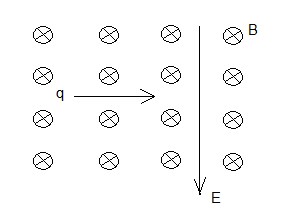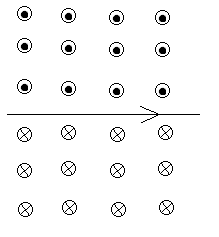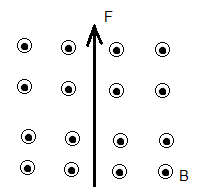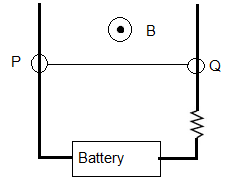# SAT Solutions to SAT Physics Practice Questions on Magnetism

 Solutions and detailed explanations to SAT Physics Practice Questions on Magnetism. NOTE: In what follows, the quantities in bold are vector quantities. A positively charged particle moves at a velocity v perpendicular to a magnetic field B. If the magnitude of the velocity v is doubled, then the magnitude of the force F acting on the moving charge is A) halved B) doubled C) quadrupled D) quartered E) not changed Solution - Explanations The magnitude of the force exerted on the particle is given by F = q v B sin(θ) = q v B sin(90 �) = q V B Let v = 2 V (double) The new force new F2 is given by F2 = q (2 V) B = 2 (q v B) = 2 F F has doubled Answer: B What is the direction of the force acting on a negatively charged particle moving from East to West in a magnetic field directed downward? A) directed to the right B) directed to the left C) directed upward D) directed into the page E) directed out of the page Solution - Explanations Using the right hand rule: index finger in the direction of motion of the particle (East to West), the middle finger in the direction of the magnetic field (downward) which gives a direction for the thumb out of the page but because the particle has a negative charge, the force will be directed into the page. Answer: D A negatively charged particle q enters from the left an area with both an electric field directed downward and a magnetic field of magnitude 6.0�10-6 T directed into the page (see figure below). What must be the magnitude of the electric field so that the particle is not deflected if the magnitude of its velocity is 2.0�105 cm/s?A) 1.2 v/m B) 3.3 � 10-11 v/m C) 1.2 � 10-2 v/m D) 3.3 � 1010 v/m E) 120 v/m Solution - Explanations On entering the area with the electric and magnetic fields, two forces are exerted on the particle. One due to the electric field and is given by q E and the second one due the magnetic field and is given by q v B, where q is the charge of the particle, E is the magnitude of the electric field, v is the magnitude of the velocity and B is the magnitude of the magnetic field. The force due to the electric field is directed upward because it is given by q E where E is a vector and the charge q is negative. The force due to the magnetic field is directed downward; using the right hand rule : index directed along the direction of motion left to right, the middle finger directed along the magnetic field into the page and the thumb will points upward but because the charge is negative, the force due to the magnetic field will be directed downward. The particle will not be deflected if the two forces have equal magnitudes since they have opposite directions. Hence q E = q v B E = v B = 2.0�105 cm/s � 6.0�10-6 T = 2.0�102 m/s � 6.0�10-6 T = 1.2 � 10-2 v/m Answer: C Which of the following diagrams represents the directions of the forces acting on the two wires with current I flowing in the same direction as shown below?Solution - Explanations First we determine the direction of the field produced by the lower wire using the right hand rule: grasp the wire with the thumb in the direction of the current and curl the fingers around the wire which gives the direction of the magnetic field produced as shown below. Above the wire the field is directed out of the page and below the wire, the field is directed into the page.We now consider the upper wire in the magnetic field produced by the lower wire. The field is directed out of the page. We now use the right hand rule to find the direction of the force F. Index finger in the direction of the current, the middle finger in the direction of the magnetic field (out of the page) and the thumb gives the direction of the force which is downward.(see diagram below)By symmetry, we can conclude that the wires will attract each other. Answer: D If a force F is exerted on a charged particle when the particle enters a magnetic field B at a velocity v, which of the following is always true? A) Vectors F, B and v are all perpendicular to each other B) Vectors F and B are parallel C) Vectors F and B are perpendicular D) Vectors F and v are be parallel E) Vectors B and v may perpendicular Solution - Explanations The force F exerted on a moving charged particle in a magnetic field is given by F = q v � B (cross product of vectors) According the properties of the cross product, F is perpendicular to both v and B. v and B may or may not be perpendicular but if they are parallel, the force is equal to zero; there is no force. Hence the only statement that is always true is when there is a force is the one in D). Answer: D If a magnetic field B exerts a force F on a wire of length L with electric current in a magnetic field, then what value of the angle between the direction of the current i and the magnetic field B gives a maximum value for the magnitude of F? A) 180� B) 45� C) 90� D) 120� E) 0 Solution - Explanations The force F exerted on a wire in a magnetic field is given by F = L i � B (cross product of vectors) The magnitude of F is given by F = L i B sin (θ) where θ is the angle between the direction of i and B. The maximum values is obtained for sin (θ) = 1 or θ = 90� Answer: C What is the direction of the current that experiences the force F of a magnetic field B shown in the diagram below?A) Upward B) into the page C) downward D) from left to right E) from right to left Solution - Explanations Apply the right hand rule: the middle finger in the direction of B (into the page), the thumb in the direction of the force F and the index gives the current from right to left. Answer: E In the diagram below, wire PQ is part of the closed circuit and is in a magnetic field B. If we neglect the resistance of the wires and the internal resistance of the battery, which of the following is true about the magnitude of the force acting on the wire PQ? (I) It is proportional to the magnitude of the magnetic field B (II) It is inversely proportional to R (III) It is inversely proportional to EA) (I) only B) (I), (II) and (III) C) (I) and (III) only D) (I) and (II) only E) (II) and (III) only Solution - Explanations The magnitude of force F on a current carrying wire PQ is given by F = L i B , i is the current in the circuit. i = E / R Hence F = L E B / R F is proportional to L, E and B and inversely proportional to R Answer: D Two parallel wires with the same current i exert forces on each other with equal magnitudes. What happens to the magnitude of this force if the current is halved? A) The magnitude is quartered B) The magnitude is halved C) The magnitude is doubled D) The magnitude is quadrupled E) The magnitude does not change Solution - Explanations The magnitude of force F on a current carrying wire of length L is given by F = L i B , i is the current in the wire , B is the magnetic field produced by the second wire. The magnitude of the magnetic field B due to a current carrying wire (second wire) is given by B = μ0 i / (2 π r) , Substitute B in F by the expression μ0 i / (2 π r) F = L B μ0 i2 / (2 π r) If you replace i by i/2 (half) then the new force is 1/4 of the force with current i. Answer: A In the circuit below wire PQ, with mass of 10 grams and length of 20 cm, can move up and down with negligible friction. If a uniform magnetic field B of magnitude 1 T and direction out of the page, is applied to wire PQ, what must be the direction and the smallest intensity of the current in the circuit that lifts wire PQ upward?A) Direction: from Q to P , Minimum intensity: 2 A B)Direction: from P to Q , Minimum intensity: 0.5 A C) Direction: from P to Q , Minimum intensity: 2 A D) Direction: from Q to P , Minimum intensity: 1 A E) Direction: from Q to P , Minimum intensity: 0.5 A Solution - Explanations The magnetic field exerts a force F on the current carrying wire PQ. For force F to lift the wire, F must be directed upward and its magnitude greater than the weight of the wire since frictions are negligible. Using the right hand rule with middle finger in the direction of B (out of the page) and the thumb in the direction of the force (up), the index is directed from right to left which gives the direction of the current (in the moving wire) from Q to P. The magnitude of force F on a current carrying wire PQ is given by F = L i B = (0.2 m) i (1 T) F > m g , m is the mass of the wire and g = 10 m/s2 is the gravitational acceleration 0.2 i > (0.01 Kg) (10 m/s2) i > 0.5 A Answer: E
More To Explore